# ME GATE 2014 - Online Test

Q1. Consider a single degree-of-freedom system with viscous damping excited by a harmonic force. At resonance, the phase angle (in degree) of the displacement with respect to the exciting force is
Explaination / Solution:
No Explaination.

Q2. The process utilizing mainly thermal energy for removing material is
Explaination / Solution:
No Explaination.

Q3. A mass m1 of 100 kg travelling with a uniform velocity of 5 m/s along a line collides with a stationary mass m2 of 1000 kg. After the collision, both the masses travel together with the same velocity. The coefficient of restitution is
Explaination / Solution: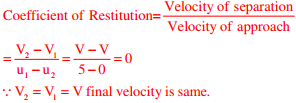Q4. A minimal spanning tree in network flow models involves
Explaination / Solution:

A path forms a loop or cycle if it connects a node itself. A spanning tree links all the nodes of network with no loops allowed.

Explaination / Solution:
No Explaination.

Q6. Which one of following is NOT correct?
Explaination / Solution:
No Explaination.

Q7. Consider a long cylindrical tube of inner and outer radii, ri and ro, respectively, length, L and thermal conductivity, k. Its inner and outer surfaces are maintained at Ti and To, respectively (Ti> To). Assuming one-dimensional steady state heat conduction in the radial direction, the thermal resistance in the wall of the tube is
Explaination / Solution: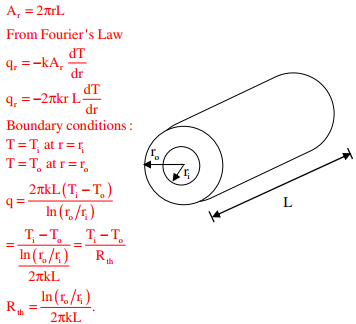Q8. Match the casting defects (Group A) with the probable causes (Group B):
Group A                                         Group B
(p) Hot tears                                  1: Improper fusion of two streams of liquid metal
(q) Shrinkage                                 2: Low permeability of the sand mould
(r) Blow holes                                3: Volumetric contraction both in liquid and solid stage
(s) Cold Shut                                 4: Differential cooling rate
Explaination / Solution:
No Explaination.

Q9. Gear 2 rotates at 1200 rpm in counter clockwise direction and engages with Gear 3. Gear 3 and Gear 4 are mounted on the same shaft. Gear 5 engages with Gear 4. The numbers of teeth on Gears 2, 3, 4 and 5 are 20, 40, 15 and 30, respectively. The angular speed of Gear 5 is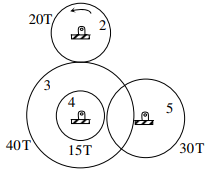Explaination / Solution: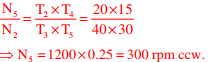Q10. Which one of the following pairs of equations describes an irreversible heat engine?
Explaination / Solution:

For clausius theorem,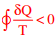(for irreversible Heat engine).